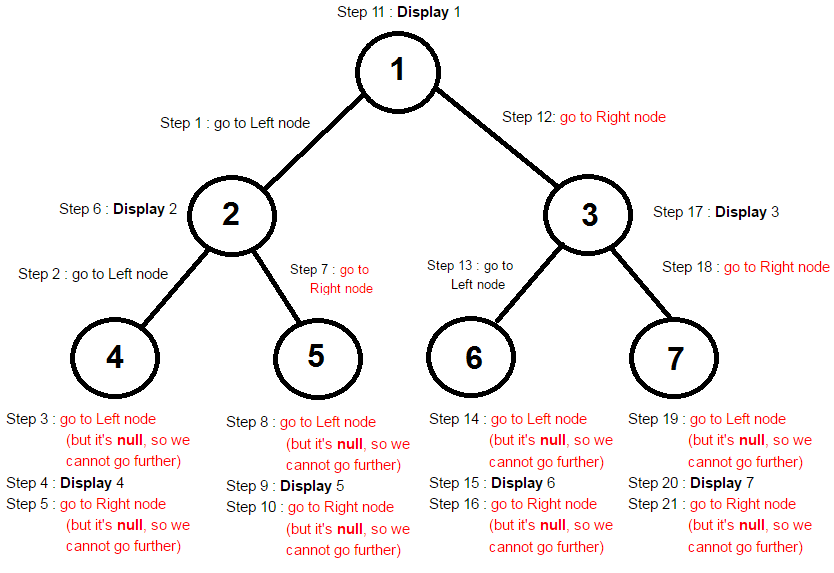### InOrder traversal of Binary Tree in java

In this data structure tutorial we will learn InOrder traversal of Binary Tree in java with program and examples.

Contents of page >
1. What happens in InOrder traversal of Binary Tree in java >
2. What are Steps in InOrder Traversal of Binary Tree in java >
3. Diagram of InOrder traversal of Binary Tree in java >
Result of InOrder traversal of Binary Tree in java is >
1. Logic for InOrder traversal of Binary Tree in java >
2. Full program/ example to show InOrder traversal of Binary Tree in java >

1. What happens in InOrder traversal of Binary Tree in java >
In InOrder traversal of Binary Tree in java first left subtree is traversed then node is processed and then right subtree is traversed.

2. What are Steps in InOrder Traversal of Binary Tree in java >
Steps in InOrder Traversal of Binary Tree in java -
1. Traverse the left subtree
2. Visit the node.
3. Traverse the right subtree.

Diagram of InOrder traversal of Binary Tree in java >Result of InOrder traversal of Binary Tree in java is >
4 2 5 1 6 3 7

4. Logic for InOrder traversal of Binary Tree in java >
 /**    * This method will do in-order Traversal of binary tree in java.    * Method will pass leftNode of binary tree recursively    * Once leftNode child is done, pass rightNode of binary tree recursively    */    public void inorderTraversalMethod(BinaryTreeNode binaryTreeNode) {           // Continue if binaryTreeNode is not null           if (binaryTreeNode != null) {                  // pass leftNode of binary tree recursively                  inorderTraversalMethod(binaryTreeNode.leftNode);                  // Display data of current binaryTreeNode.                  System.out.print(binaryTreeNode.data + " ");                  // pass rightNode of binary tree recursively                  inorderTraversalMethod(binaryTreeNode.rightNode);           }    }

5. Full program/ example to show InOrder traversal of Binary Tree in java >
 /** * www.JavaMadeSoEasy.com * Write a program to perform InOrder Traversal of Binary Tree in java * * Class to perform InOrder Traversal of Binary Tree in java */ public class InOrderTraversalOfBinaryTree {       /**    * Create Node of Binary Tree    */    public static class BinaryTreeNode {           // Left and right node of Binary tree           BinaryTreeNode leftNode;           BinaryTreeNode rightNode;           // Data of BinaryTree           int data;           BinaryTreeNode(int data) {                  this.data = data;           }    }    /**    * This method will do in-order Traversal of binary tree in java.    * Method will pass leftNode of binary tree recursively    * Once leftNode child is done, pass rightNode of binary tree recursively    */    public void inorderTraversalMethod(BinaryTreeNode binaryTreeNode) {           // Continue if binaryTreeNode is not null           if (binaryTreeNode != null) {                  // pass leftNode of binary tree recursively                  inorderTraversalMethod(binaryTreeNode.leftNode);                  // Display data of current binaryTreeNode.                  System.out.print(binaryTreeNode.data + " ");                  // pass rightNode of binary tree recursively                  inorderTraversalMethod(binaryTreeNode.rightNode);           }    }    /**    * Main method to perform InOrder Traversal of Binary Tree in java    */    public static void main(String[] args) {           InOrderTraversalOfBinaryTree inOrderTraversalOfBinaryTree =                                                    new InOrderTraversalOfBinaryTree();           // Now, let's start creating nodes of Binary tree           // create node1 (which is ROOT node)           BinaryTreeNode binaryTreeNode1 = new BinaryTreeNode(1);           // Left node of node1           BinaryTreeNode node2 = new BinaryTreeNode(2);           binaryTreeNode1.leftNode = node2;           // Right node of node1           BinaryTreeNode node3 = new BinaryTreeNode(3);           binaryTreeNode1.rightNode = node3;           // Left node of node2           BinaryTreeNode node4 = new BinaryTreeNode(4);           node2.leftNode = node4;           // Right node of node2           BinaryTreeNode node5 = new BinaryTreeNode(5);           node2.rightNode = node5;           // Left node of node3           BinaryTreeNode node6 = new BinaryTreeNode(6);           node3.leftNode = node6;           // Right node of node3           BinaryTreeNode node7 = new BinaryTreeNode(7);           node3.rightNode = node7;           System.out.println("In order Traversal of Binary Tree in java : ");           // Pass root node to in order traversal method           inOrderTraversalOfBinaryTree.inorderTraversalMethod(binaryTreeNode1);    } } /* Output In order Traversal of Binary Tree in java : 4 2 5 1 6 3 7 */

Summary -
So in this data structure tutorial we learned In InOrder traversal of Binary Tree in java first left subtree is traversed then node is processed and then right subtree is traversed. Steps in InOrder Traversal of Binary Tree in java. Diagram of InOrder traversal of Binary Tree in java.Logic for InOrder traversal of Binary Tree in java. Full program/ example to show InOrder traversal of Binary Tree in java.

Having any doubt? or you liked the tutorial! Please comment in below section.